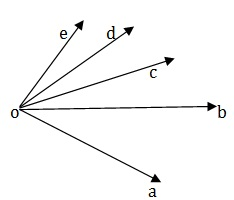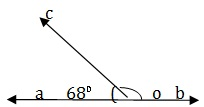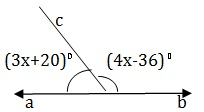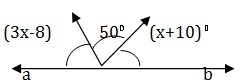# Geometry - Solved Examples

Explanation

```A line has no points.
```

Explanation

```A line segment has two end points.
```

Explanation

```A ray has one end point.
```

Q 4 - An angle which is greater then 180° but less than 360° is called

A - Acute Angle

B - Obtuse Angle

C - Straight Angle

D - Reflex Angle

Explanation

```An angle which is greater than 180°  but less than 360° is called a reflex angle.
```

Q 5 - The complement of 62° is.

A - 118°

B - 28°

C - 38°

D - 48°

Explanation

```Complement of 62°= (90° – 62°) = 28°.
```

Q 6 - The supplement of 60° is

A - 30°

B - 40°

C - 120°

D - 300°

Explanation

```Supplement of 60° = (180°-60°) =120°.
```

Q 7 - The complement of 72° 40' is

A - 107°20'

B - 27°20'

C - 17°20'

D - 12°40'

Explanation

```Complement of 72° 40' = (90°-72° 40') =17° 20'.
```

Q 8 - An angle is one fifth of its supplement. The measure of the angle is

A - 15°

B - 30°

C - 75°

D - 150°

Explanation

```x = 1/5 (180 – x )⇒ 5x = 180 – x ⇒ 6x = 180 ⇒ x = 30°.
```

Q 9 - If an angle is its own complementary angle, then its measure is

A - 30°

B - 45°

C - 60°

D - 90°

Explanation

```x=(90-x) ⇒ 2x = 90 ⇒ x = 45° .
```

Q 10 - How many angles are made by rays shown in the figure?A - 5

B - 6

C - 8

D - 10

Explanation

```The angle are ∠AOB , ∠BOC,∠COD,∠DOE,∠AOC,∠AOD, ∠AOE,∠BOD,∠BOD,∠COE.
Thus , 10 angle are  formed.
```

Q 11 - An angle is 24° more than its complement.The measure of the angle is

A - 57°

B - 47°

C - 53°

D - 66°

Explanation

```x – (90-x ) = 24 ⇒ 2x = 114 ⇒ x = 57
∴ Required angle is 57°.
```

Q 12 -An angle is 32° less than its supplement. The measure of the angle is

A - 37°

B - 74°

C - 48°

D - 66°

Explanation

```(180 –X) – X = 32 ⇒  2x = 180 – 32 = 148 ⇒ x = 74.
Required angle is 74°.
```

Q 13 - Two Supplementary angles are in th ratio 3:2. The smaller angle measures

A - 108°

B - 81°

C - 72°

D - 66°

Explanation

```Let the measures of the angle be (3x)° and (2x)°. Then,
3x+2x=180 ⇒ 5x = 180 ⇒ x = 36.
Smaller angle = (2x)° = (2*36)° = 72°.
```

Q 14 - In the given figure, AOB is a straight line, ∠AOC = 68° and ∠BOC = x°. The value of the x isA - 120°

B - 22°

C - 112°

D - 132°

Explanation

```Since ∠AOB is a straight angle , we have
X+ 68 = 180 ⇒ x= (180-68)°  = 120°
```

Q 15 - In the given figure , AOB is a straight line, ∠AOC = (3x+20)° and ∠ BOC =(4 x-36)°. The value of the x isA - 32°

B - 22°

C - 26°

D - 24°

Explanation

```Since ∠AOB is a straight angle , we have
∠AOC + ∠ BOC  =180°
⇒ 3x + 20 +4x – 36 = 180
⇒ 7x = 164 ⇒ x = 22.
```

Q 16 - In the given figure , AOB is a straight line, ∠ AOC = (3x-8)° and ∠COD =50 and ∠BOD° =(x+10)°. The value of the x isA - 32°

B - 42°

C - 36°

D - 52°

```Since ∠AOB is a straight angle , we have